# Grade 3 Data and Graphing Worksheets

## Draw and analyze graphs

Our grade 3 data and graphing worksheets expand on early data collection, graphing and analyses skills. In particular, scaled graphs and more complex data sets are introduced.

 Graphing Tally Counts Make graphs from tally counts.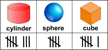Spin, Tally, Graph & Analyze Create and graph randomized data.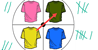Scaled Pictographs Analyze pictographs where symbols represent multiple items.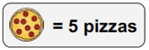Scaled Bar Graphs Draw and analyze scaled bar graphs.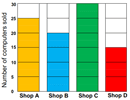Analyzing Scaled Bar Graphs Analyze scaled bar graphs without referring to a data table. Interpreting Line Plots Interpret line plots without referring to a data table.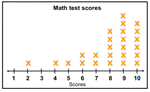Making Line Plots Create and analyze line plots from a data set. Circle Graphs Create and analyze circle graphs (with / without fractions).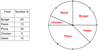Data collection Collect (survey, observe) data and graph it.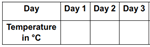Venn Diagrams Analyze double and triple Venn diagrams.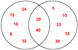Line Graphs Draw and analyze line graphs.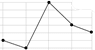Labelling Graphs Scale and label graphs.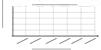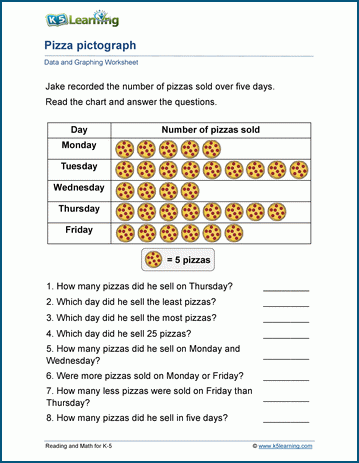Sample grade 3 data & graphing worksheet

What is K5?

K5 Learning offers free worksheets, flashcards and inexpensive workbooks for kids in kindergarten to grade 5. Become a member to access additional content and skip ads.